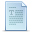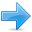# Name visibility

### Scopes

Named entities, such as variables, functions, and compound types need to be declared before being used in C++. The point in the program where this declaration happens influences its visibility:

An entity declared outside any block has global scope, meaning that its name is valid anywhere in the code. While an entity declared within a block, such as a function or a selective statement, has block scope, and is only visible within the specific block in which it is declared, but not outside it.

Variables with block scope are known as local variables.

For example, a variable declared in the body of a function is a local variable that extends until the end of the the function (i.e., until the brace `}` that closes the function definition), but not outside it:

 ``12345678910111213`` ``````int foo; // global variable int some_function () { int bar; // local variable bar = 0; } int other_function () { foo = 1; // ok: foo is a global variable bar = 2; // wrong: bar is not visible from this function }``````

In each scope, a name can only represent one entity. For example, there cannot be two variables with the same name in the same scope:

 ``1234567`` ``````int some_function () { int x; x = 0; double x; // wrong: name already used in this scope x = 0.0; }``````

The visibility of an entity with block scope extends until the end of the block, including inner blocks. Nevertheless, an inner block, because it is a different block, can re-utilize a name existing in an outer scope to refer to a different entity; in this case, the name will refer to a different entity only within the inner block, hiding the entity it names outside. While outside it, it will still refer to the original entity. For example:

 ``````// inner block scopes #include using namespace std; int main () { int x = 10; int y = 20; { int x; // ok, inner scope. x = 50; // sets value to inner x y = 50; // sets value to (outer) y cout << "inner block:\n"; cout << "x: " << x << '\n'; cout << "y: " << y << '\n'; } cout << "outer block:\n"; cout << "x: " << x << '\n'; cout << "y: " << y << '\n'; return 0; }`````` ```inner block: x: 50 y: 50 outer block: x: 10 y: 50```

Note that `y` is not hidden in the inner block, and thus accessing `y` still accesses the outer variable.

Variables declared in declarations that introduce a block, such as function parameters and variables declared in loops and conditions (such as those declared on a for or an if) are local to the block they introduce.

### Namespaces

Only one entity can exist with a particular name in a particular scope. This is seldom a problem for local names, since blocks tend to be relatively short, and names have particular purposes within them, such as naming a counter variable, an argument, etc...

But non-local names bring more possibilities for name collision, especially considering that libraries may declare many functions, types, and variables, neither of them local in nature, and some of them very generic.

Namespaces allow us to group named entities that otherwise would have global scope into narrower scopes, giving them namespace scope. This allows organizing the elements of programs into different logical scopes referred to by names.

The syntax to declare a namespaces is:

 ``` namespace identifier { named_entities } ```

Where `identifier` is any valid identifier and `named_entities` is the set of variables, types and functions that are included within the namespace. For example:

 ``1234`` ``````namespace myNamespace { int a, b; }``````

In this case, the variables `a` and `b` are normal variables declared within a namespace called `myNamespace`.

These variables can be accessed from within their namespace normally, with their identifier (either `a` or `b`), but if accessed from outside the `myNamespace` namespace they have to be properly qualified with the scope operator `::`. For example, to access the previous variables from outside `myNamespace` they should be qualified like:

 ``12`` ``````myNamespace::a myNamespace::b``````

Namespaces are particularly useful to avoid name collisions. For example:

 ``````// namespaces #include using namespace std; namespace foo { int value() { return 5; } } namespace bar { const double pi = 3.1416; double value() { return 2*pi; } } int main () { cout << foo::value() << '\n'; cout << bar::value() << '\n'; cout << bar::pi << '\n'; return 0; }`````` ```5 6.2832 3.1416```

In this case, there are two functions with the same name: `value`. One is defined within the namespace `foo`, and the other one in `bar`. No redefinition errors happen thanks to namespaces. Notice also how `pi` is accessed in an unqualified manner from within namespace `bar` (just as `pi`), while it is again accessed in `main`, but here it needs to be qualified as `bar::pi`.

Namespaces can be split: Two segments of a code can be declared in the same namespace:

 ``123`` ``````namespace foo { int a; } namespace bar { int b; } namespace foo { int c; }``````

This declares three variables: `a` and `c` are in namespace `foo`, while `b` is in namespace `bar`. Namespaces can even extend across different translation units (i.e., across different files of source code).

### using

The keyword `using` introduces a name into the current declarative region (such as a block), thus avoiding the need to qualify the name. For example:

 ``````// using #include using namespace std; namespace first { int x = 5; int y = 10; } namespace second { double x = 3.1416; double y = 2.7183; } int main () { using first::x; using second::y; cout << x << '\n'; cout << y << '\n'; cout << first::y << '\n'; cout << second::x << '\n'; return 0; }`````` ```5 2.7183 10 3.1416```

Notice how in `main`, the variable `x` (without any name qualifier) refers to `first::x`, whereas `y` refers to `second::y`, just as specified by the `using` declarations. The variables `first::y` and `second::x` can still be accessed, but require fully qualified names.

The keyword `using` can also be used as a directive to introduce an entire namespace:
 ``````// using #include using namespace std; namespace first { int x = 5; int y = 10; } namespace second { double x = 3.1416; double y = 2.7183; } int main () { using namespace first; cout << x << '\n'; cout << y << '\n'; cout << second::x << '\n'; cout << second::y << '\n'; return 0; }`````` ```5 10 3.1416 2.7183```

In this case, by declaring that we were using namespace `first`, all direct uses of `x` and `y` without name qualifiers were also looked up in namespace `first`.

`using` and `using namespace` have validity only in the same block in which they are stated or in the entire source code file if they are used directly in the global scope. For example, it would be possible to first use the objects of one namespace and then those of another one by splitting the code in different blocks:

 ``````// using namespace example #include using namespace std; namespace first { int x = 5; } namespace second { double x = 3.1416; } int main () { { using namespace first; cout << x << '\n'; } { using namespace second; cout << x << '\n'; } return 0; }`````` ```5 3.1416```

### Namespace aliasing

Existing namespaces can be aliased with new names, with the following syntax:

`namespace new_name = current_name;`

### The std namespace

All the entities (variables, types, constants, and functions) of the standard C++ library are declared within the `std` namespace. Most examples in these tutorials, in fact, include the following line:

 ``1`` ``using namespace std;``

This introduces direct visibility of all the names of the `std` namespace into the code. This is done in these tutorials to facilitate comprehension and shorten the length of the examples, but many programmers prefer to qualify each of the elements of the standard library used in their programs. For example, instead of:

 ``1`` ``cout << "Hello world!";``

It is common to instead see:

 ``1`` ``std::cout << "Hello world!";``

Whether the elements in the `std` namespace are introduced with `using` declarations or are fully qualified on every use does not change the behavior or efficiency of the resulting program in any way. It is mostly a matter of style preference, although for projects mixing libraries, explicit qualification tends to be preferred.

### Storage classes

The storage for variables with global or namespace scope is allocated for the entire duration of the program. This is known as static storage, and it contrasts with the storage for local variables (those declared within a block). These use what is known as automatic storage. The storage for local variables is only available during the block in which they are declared; after that, that same storage may be used for a local variable of some other function, or used otherwise.

But there is another substantial difference between variables with static storage and variables with automatic storage:
- Variables with static storage (such as global variables) that are not explicitly initialized are automatically initialized to zeroes.
- Variables with automatic storage (such as local variables) that are not explicitly initialized are left uninitialized, and thus have an undetermined value.

For example:
 ``````// static vs automatic storage #include using namespace std; int x; int main () { int y; cout << x << '\n'; cout << y << '\n'; return 0; }`````` ```0 4285838```

The actual output may vary, but only the value of `x` is guaranteed to be zero. `y` can actually contain just about any value (including zero).Previous:Overloads and templatesIndexNext:Arrays